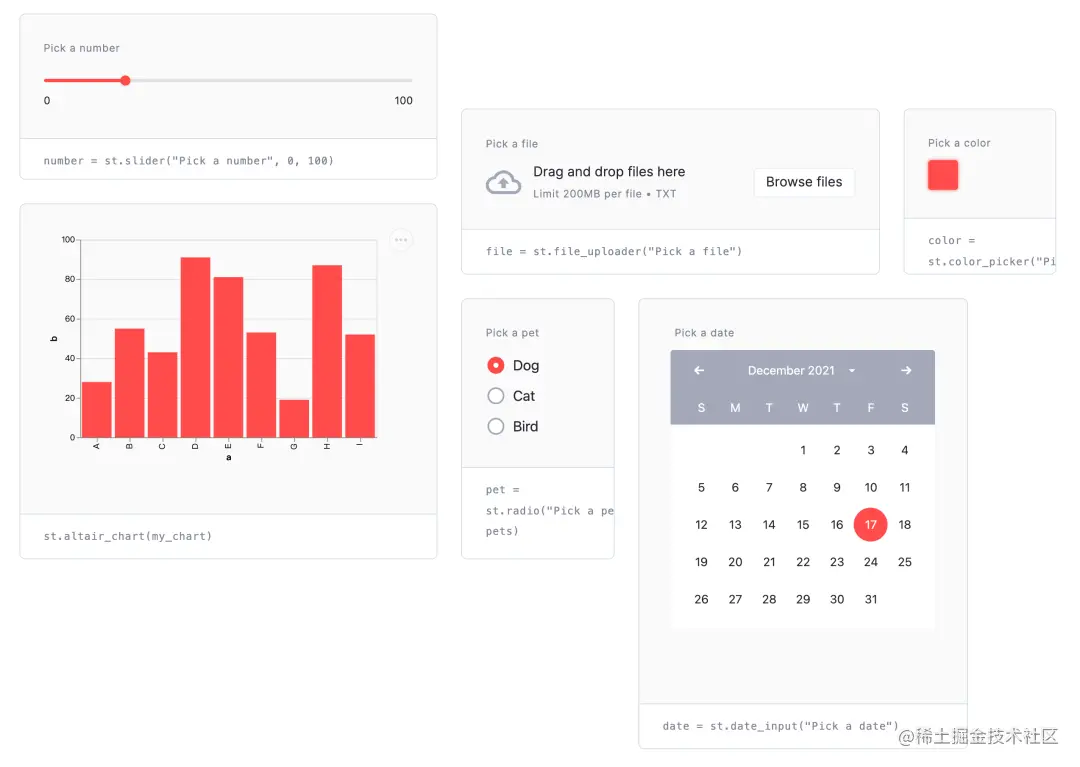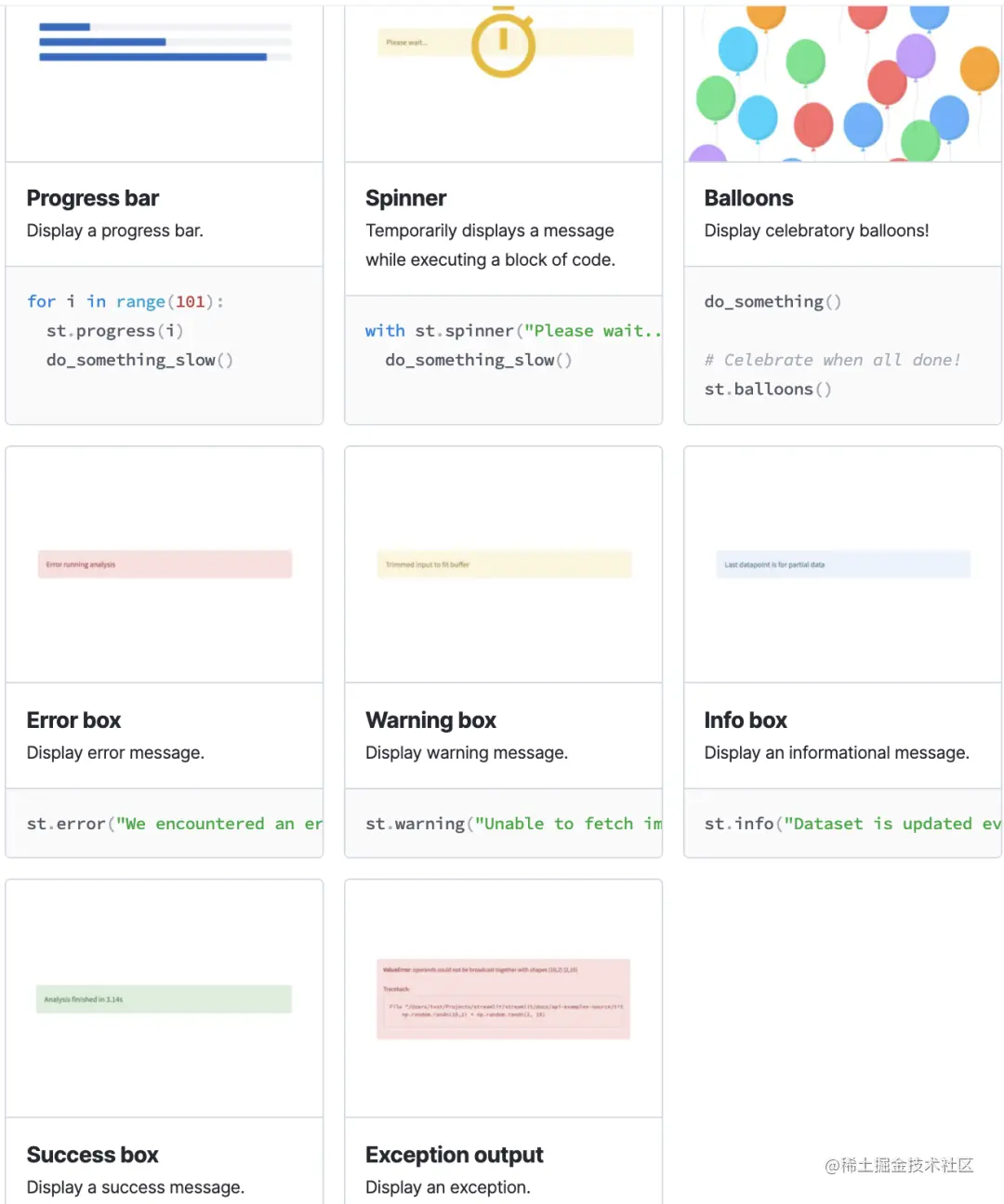﻿ 构建可视化 web的 Python 神器streamlit_python_脚本之家
python# 构建可视化 web的 Python 神器streamlit

Streamlit 是一个用于机器学习、数据可视化的 Python 框架，它能几行代码就构建出一个精美的在线 app 应用。

## 1. 如何安装？

`➜ pip install streamlit `

`➜ python3 -m venv .`

`➜ source ./venv/bin/activate`

```➜ pip list | wc -l
92```

## 2. 入门示例

Streamlit 提供了一些入门示例，执行如下命令即可

`➜ streamlit hello``➜ streamlit run st-demo.py`

## 2. Markdown 文本

• st.title()：文章大标题
• st.text()：文本
• st.code()：代码，同时可设置代码的语言，显示的时候会高亮
• st.latex()：latex 公式
• st.caption()：小字体文本

```import streamlit as st

# markdown
st.markdown('Streamlit Demo')

# 设置网页标题
st.title('一个傻瓜式构建可视化 web的 Python 神器 -- streamlit')

# 展示一级标题

st.text('和安装其他包一样，安装 streamlit 非常简单，一条命令即可')
code1 = '''pip3 install streamlit'''
st.code(code1, language='bash')

# 展示一级标题

# 展示二级标题

# 纯文本
st.text('导入 streamlit 后，就可以直接使用 st.markdown() 初始化')

# 展示代码，有高亮效果
code2 = '''import streamlit as st
st.markdown('Streamlit Demo')'''
st.code(code2, language='python')```

Streamlit 运行的方式 与普通的脚本 有所不同，应该使用 `streamlit run st-demo.py`## 3. 数据图表支持

### 3.1 图表组件

• table：普通的表格，用于静态数据的展示
• dataframe：高级的表格，可以进行数据的操作，比如排序

Table 的示例：

```df = pd.DataFrame(
np.random.randn(10, 5),
columns=('第%d列' % (i+1) for i in range(5))
)

st.table(df)```Datafram 的示例：

```df = pd.DataFrame(
np.random.randn(10, 5),
columns=('第%d列' % (i+1) for i in range(5))
)

st.dataframe(df.style.highlight_max(axis=0))```• highlight_null：空值高亮
• highlight_min：最小值高亮
• highlight_max：最大值高亮
• highlight_between：某区间内的值高亮
• highlight_quantile：暂没用过

### 3.2 监控组件

```col1, col2, col3 = st.columns(3)
col1.metric("Temperature", "70 °F", "1.2 °F")
col2.metric("Wind", "9 mph", "-8%")
col3.metric("Humidity", "86%", "4%")```### 3.3 原生图表组件

Streamlit 原生支持多种图表：

• st.line_chart：折线图
• st.area_chart：面积图
• st.bar_chart：柱状图
• st.map：地图

```chart_data = pd.DataFrame(
np.random.randn(20, 3),
columns=['a', 'b', 'c'])
st.line_chart(chart_data)``````chart_data = pd.DataFrame(
np.random.randn(20, 3),
columns = ['a', 'b', 'c'])
st.area_chart(chart_data)``````chart_data = pd.DataFrame(
np.random.randn(50, 3),
columns = ["a", "b", "c"])
st.bar_chart(chart_data)``````df = pd.DataFrame(
np.random.randn(1000, 2) / [50, 50] + [37.76, -122.4],
columns=['lat', 'lon']
)
st.map(df)```### 3.4 外部图表组件

Streamlit 的一些原生图表组件，虽然做到了傻瓜式，但仅能输入数据、高度和宽度，如果你想更漂亮的图表，就像 matplotlib.pyplot、Altair、vega-lite、Plotly、Bokeh、PyDeck、Graphviz 那样，streamlit 也提供了支持：

• st.pyplot
• st.bokeh_chart
• st.altair_chart
• st.altair_chart
• st.vega_lite_chart
• st.plotly_chart
• st.pydeck_chart
• st.graphviz_chart

## 4. 用户操作支持

• button：按钮
• checkbox：复选框
• selectbox：下拉单选框
• multiselect：下拉多选框
• slider：滑动条
• select_slider：选择条
• text_input：文本输入框
• text_area：文本展示框
• number_input：数字输入框，支持加减按钮
• date_input：日期选择框
• time_input：时间选择框
• color_picker：颜色选择器## 5. 多媒体组件

• st.image
• st.audio
• st.video## 6. 状态组件

• progress：进度条，如游戏加载进度
• spinner：等待提示
• balloons：页面底部飘气球，表示祝贺
• error：显示错误信息
• warning：显示报警信息
• info：显示常规信息
• success：显示成功信息
• exception：显示异常信息（代码错误栈）## 7. 页面布局

Streamlit 是自上而下渲染的，组件在页面上的排列顺序与代码的执行顺序一致。

st.sidebar：侧边栏• st.columns：列容器，处在同一个 columns 内组件，按照从左至右顺序展示
• st.expander：隐藏信息，点击后可展开展示详细内容，如：展示更多
• st.container：包含多组件的容器
• st.empty：包含单组件的容器

## 8. 流程控制系统

Streamlit 是自上而下逐步渲染出来的，若你的应用场景需要对渲染做一些控制，streamlit 也有提供对应的方法

• st.stop：可以让 Streamlit 应用停止而不向下执行，如：验证码通过后，再向下运行展示后续内容。
• st.form：表单，Streamlit 在某个组件有交互后就会重新执行页面程序，而有时候需要等一组组件都完成交互后再刷新（如：登录填用户名和密码），这时候就需要将这些组件添加到 form 中
• st.form_submit_button：在 form 中使用，提交表单。

## 9. 缓存特性提升速度

```DATE_COLUMN = 'date/time'
DATA_URL = ('https://s3-us-west-2.amazonaws.com/'
'streamlit-demo-data/uber-raw-data-sep14.csv.gz')

@st.cache
lowercase = lambda x: str(x).lower()
data.rename(lowercase, axis='columns', inplace=True)
data[DATE_COLUMN] = pd.to_datetime(data[DATE_COLUMN])
return data```

## 10. 部署上线• requirements.txt：依赖包文件
• setup.sh：安装脚本，主要是创建文件夹，写入配置文件
• Procfile：启动脚本，告诉 Heroku 如何安装并启动应用```git init
git commit -m "init"```

```heroku login
heroku create
git push heroku master
heroku ps:scale web=1```

`heroku logs --tail`

`heroku domains:add hivecnstats.iswbm.com`

## 12. 总结

Streamlit 一个开箱即用的工具集，它可以让一个普通的个人开发者免于学习繁杂的前端知识，就可以轻松、快速的构建一个简洁、优雅的 web app 应用，这是 streamlit 最吸引人的地方。